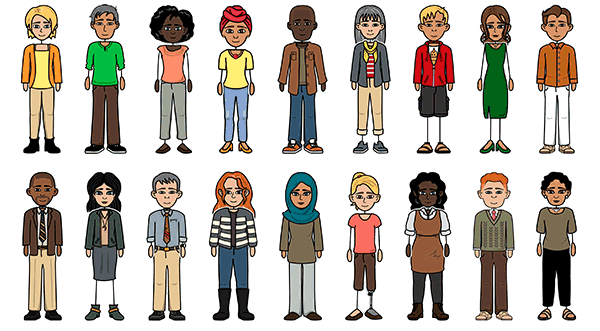# Roller Coaster Tycoon

Create a Storyboard#### Storyboard Text

• 1. Hi, I am Jaanbir.
• 3. Okay.
• 2. Hi, I am Jasnoor, the owner of this Amusement park. Can you help me figure out my expenses, revenue, and how to make profit?
• 1. What are the total variable expenses per visitor? What about the fixed costs?
• 3. 15x + 17,000 is an expression that represents the total expenses. X represents the number of visitors you will have, while 15 is the total variable expense per visitor and 17,000 is the total fixed cost for the park, like you said.
• 2. To begin with, the total variable expenses per visitor is \$15. The total fixed cost for the park is \$17,000.
• 1. What is the total variable revenues per person? And the total fixed revenues?
• 3. Yes! That's correct! X is still representing the number of visitors, and it is being multiplied by each of their costs, which is 83. The 2,500 represents the total fixed revenues addition. Now, all that is left is coming up with an inequality representing your profit!
• 2. 83 total variable revenues per person, and 2,500 total fixed revenues. I'm assuming the expression will be 83x + 2,500?
• *Thinking* Hm...Oh yes! In order to make profit, the inequality should be83x + 2,500 15x + 17,000.
• 1. Now, let's solve the inequality. 83x + 2,500 15x + 17,000. First, let's get all X's to one side by subtracting 15x from itself on one side, and then from 83x on the other side. Then we are left with the inequality: 68x + 2,500 17,000. Then, we can continue solving it as we would a normal inequality.
• 3. X 214! This means that in order to make a profit, more than 214 visitors are required daily.
• 2. Subtract 2,500 from itself, since it's positive and then do the same to 17,000, since both sides need to be treated equally! After that, we are left with 68x 17,000. Divide 68 on both sides to isolate the variable and to get our answer, which would be...
• 1. Here is the math:
• 4. No problem! That was fun. Bye!
• 3. Thanks for your help! Bye!
• 2. Here is the inequality's answer graphed:
Over 30 Million Storyboards Created